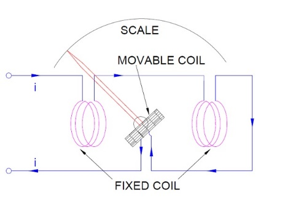Engineering Jobs   »   uprvunl je electrical quiz

# UPRVUNL’21 EE: Daily Practices Quiz 28-July-2021

Each question carries 1 mark.
Negative marking: 1/4 mark
Total Questions: 06
Time: 08 min.

Q1. The operating voltage for low tension (LT) cables is up to
(a) 220 V
(b) 400 V
(c) 1000 V
(d) None of the above

Q2. In a series resonant circuit, at resonance, selectivity Q is equal to
(a) 1/(R√LC)
(b) 1/R √(C/L)
(c) 1/R √(L/C)
(d) 1/R √LC

Q3. Which among the following equation is true for a steady magnetic field?
Where B is magnetic flux density, J is current density and H is magnetic field intensity.
(a) ∇⋅B = J
(b) ∇ ×H = 0
(c) ∇ ×H = J
(d) None of the above

Q4. A transformer is working at its full load and its efficiency is also maximum. The iron loss is 500 watts. Then, its copper loss at half of full load will be:
(a) 500 watts
(b) 250 watts
(c) 125 watts
(d) 200 watts

Q5. If the full load copper loss and iron loss of a transformer are 200 W and 100 W respectively. Their corresponding value at half load will be respectively:
(a) 200 W and 100 W
(b) 100 W and 100 W
(c) 200 W and 25 W
(d) 50 W and 100 W

Q6. The moving coil in a dynamometer is connected
(a) In series with the fixed coil
(b) Across the supply
(c) In series with the load

SOLUTIONS

S1. Ans.(c)
Sol. LT cables are meant for use up to 1000 V.

S2. Ans.(c)
Sol. for series resonance, Quality factor(Q)=1/R √(L/C)

S3. Ans.(c)
Sol. From Maxwell’s second law: ∇ ×H = J+∂D/∂t
∵ For steady magnetic field: ∇ ×H = J

S4. Ans.(c)
Sol. The transformer is working at its full load and its efficiency is also maximum.
So, iron loss=copper loss=500 watts

S5. Ans.(d)
Sol. In a transformer, copper loss depends on load.